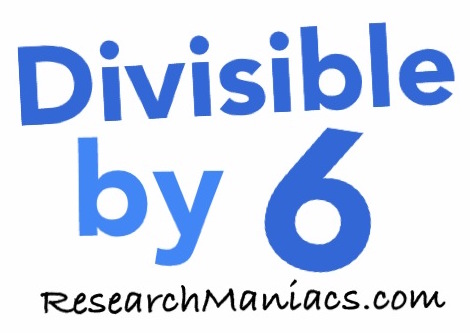Is 10 divisible by 6?The question we are solving here is: If we divide 10 by 6, will we get a whole number with no remainder? In other words, is 10 divisible by 6?

For 10 to be divisible by 6, both of the following two criteria must be met: First, 10 has to be an even number and second, the sum of its digits must be divisible by 3.

Looking at 10, you see that 10 is an even number, which means that the first criteria is met.

The sum of the digits in 10 is 1, which is not divisible by 3. Therefore, the second criteria is not met.

Thus, 10 is not divisible by 6 and the answer to the question "Is 10 divisible by 6?" is

NO

You may also be interested to know that if you divide 10 by 6, you get 1.67.

Divisible by 6?
Do you need to check another number? Enter a number below to see if it is divisible by 6.

Is 11 divisible by 6?
Can you do it on your own now? If not, go here for the next number on our list.